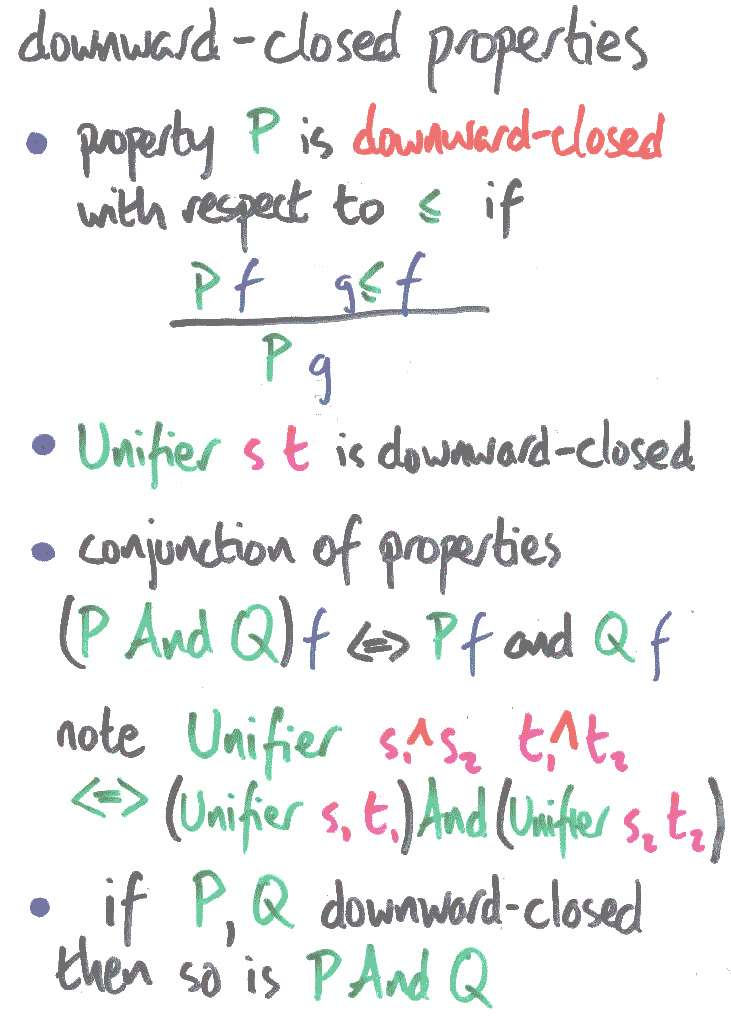Next: the optimist's theorem | Prev: accumulating | Up: contentsWhat makes the accumulator technique work? Think of the problem decomposed into the constraint which remains to be solved and the constraints we've solved already. The latter are represented in a BMax property solely by the bound b, so we require that the `constraints so far' be reducible to a single bounding constraint.

Hence, in particular, we require that once we have found a satisfactory arrow within the bound, any smaller arrow is also a solution. That is, the `constraints so far' must be `downward-closed'. Downward closure is a bit like the convexity condition in more traditional optimisation scenarios.

Note that being a unifier is a downward-closed property. The `like-constructors' cases of unification decompose a single equation into a conjunction of several, by injectivity. Fortunately the conjunction of downward-closed properties is downward-closed.

This decomposition gives us a simple strategy for solving the problem: `optimisation by optimism'. We may decompose the problem into smaller and smaller bits which we solve in sequence, relative to an accumulating guess at the solution. This guess is always optimistic, initially the top element, and we only reduce it reluctantly, when an atomic constraint forces us to accept reality.

Claudio Russo points out that finding the biggest number in a list is a nice simple example of this technique.

Let's check that this really works.

Next: the optimist's theorem | Prev: accumulating | Up: contents

Conor's Work Page